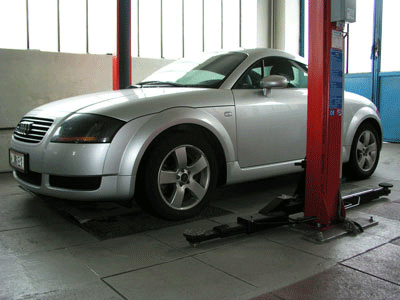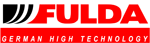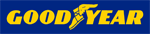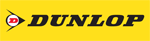F L E X I B L E    A B H O L U N G - R E P A R A T U R A N N A H M E A U S S E R H A L B    D E R    Ö F F N U N G S Z E I T E N N A C H    T E R M I N V E R E I N B A H R U N G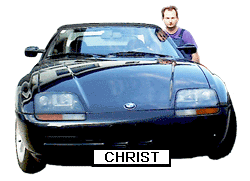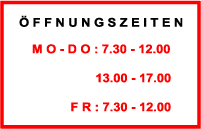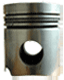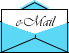W I R   F R E U E N   U N S   A U F   I H R E N   B E S U C H

C H R I S T     W A G R A M E R S T R A S S E     1 8 9 a     A - 1 2 1 0   W I E N